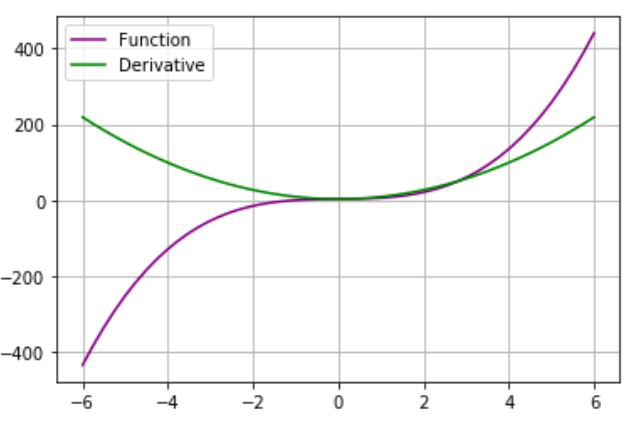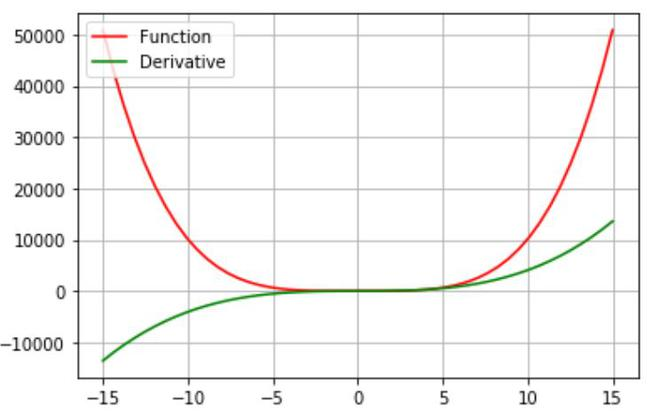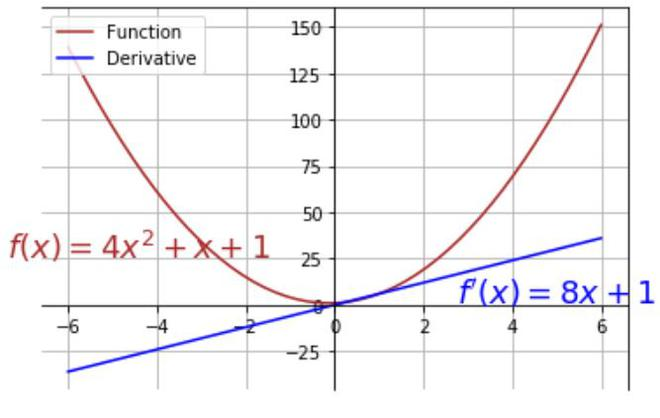# How to calculate and plot the derivative of a function using Python – Matplotlib ?

• Difficulty Level : Medium
• Last Updated : 24 Feb, 2021

In this article, we will plot the derivative of a function using matplotlib and python. For this we are using some modules in python which are as follows:

• Matplotlib: Matplotlib is one of the most popular Python packages used for data visualization. It is a cross-platform library for making 2D plots from data in arrays.
• NumPy: It is a python library that is used for working with arrays, it also supports large multi-dimensional arrays and matrices, it also has several mathematical functions.
• SciPy: Python has a library named as SciPy that is used for mathematical, scientific, and engineering calculations. This library depends on NumPy, and provides various numerical operations.

To plot the derivative of a function first, we have to calculate it. The scipy.misc library has a derivative() function which accepts one argument as a function and the other is the variable w.r.t which we will differentiate the function. So we will make a method named function() that will return the original function and a second method named deriv() that will return the derivative of that function.

Attention geek! Strengthen your foundations with the Python Programming Foundation Course and learn the basics.

To begin with, your interview preparations Enhance your Data Structures concepts with the Python DS Course. And to begin with your Machine Learning Journey, join the Machine Learning - Basic Level Course

After this calculation of the derivative of the input function, we will use the NumPy linspace() function which sets the range of the x-axis. The plot() function will be used to plot the function and also the derivative of that function.

Approach:

• Import the modules required.
• Define methods for function and its derivative
• Use NumPy linspace function to make x-axis spacing.
• Plot the function and its derivative
• Change the limits of axis using gca() function
• Plot the text using text() function

Example 1: (Derivative of cubic)

In this example, we will give the function f(x)=2x3+x+3 as input, then calculate the derivative and plot both the function and its derivative.

## Python3

 `# importing the library``import` `matplotlib.pyplot as plt``from` `scipy.misc ``import` `derivative``import` `numpy as np`` ` `# defining the function``def` `function(x):``    ``return` `2``*``x``*``x``*``x``+``x``+``3`` ` `# calculating its derivative``def` `deriv(x):``    ``return` `derivative(function, x)`` ` `# defininf x-axis intervals``y ``=` `np.linspace(``-``6``, ``6``)`` ` `# plotting the function``plt.plot(y, function(y), color``=``'purple'``, label``=``'Function'``)`` ` `# plotting its derivative``plt.plot(y, deriv(y), color``=``'green'``, label``=``'Derivative'``)`` ` `# formatting``plt.legend(loc``=``'upper left'``)``plt.grid(``True``)`

Output:Example 2: (Derivative of Poly degree polynomial)

In this example, we will give the function f(x)=x4+x2+5 as input, then calculate the derivative and plot both the function and its derivative.

## Python3

 `# importing the library``import` `matplotlib.pyplot as plt``from` `scipy.misc ``import` `derivative``import` `numpy as np`` ` `# defining the function``def` `function(x):``    ``return` `x``*``x``*``x``*``x``+``x``*``x``+``5`` ` `# calculating its derivative``def` `deriv(x):``    ``return` `derivative(function, x)`` ` ` ` `# defininf x-axis intervals``y ``=` `np.linspace(``-``15``, ``15``)`` ` `# plotting the function``plt.plot(y, function(y), color``=``'red'``, label``=``'Function'``)`` ` `# plotting its derivative``plt.plot(y, deriv(y), color``=``'green'``, label``=``'Derivative'``)`` ` `# formatting``plt.legend(loc``=``'upper left'``)``plt.grid(``True``)`

Output:Example 3: (Derivative of quadratic with formatting by text)

In this example, we will plot the derivative of f(x)=4x2+x+1. Also, we will use some formatting using the gca() function that will change the limits of the axis so that both x, y axes intersect at the origin. The text() function which comes under matplotlib library plots the text on the graph and takes an argument as (x, y) coordinates. We will also do some formatting.

## Python3

 `# importing modules``import` `matplotlib.pyplot as plt``from` `scipy.misc ``import` `derivative``import` `numpy as np`` ` `# method to return function``def` `function(x):``    ``return` `4``*``x``*``*``2``+``x``+``1`` ` `# method to return its derivative``def` `deriv(x):``    ``return` `derivative(function, x)`` ` `#range in x-axis``y ``=` `np.linspace(``-``6``, ``6``)`` ` `# plotting function``plt.plot(y, function(y), color``=``'brown'``, label``=``'Function'``)`` ` `# plotting its derivative``plt.plot(y, deriv(y), color``=``'blue'``, label``=``'Derivative'``)`` ` `# changing limits of y-axis``plt.gca().spines[``'left'``].set_position(``'zero'``,)`` ` `# changing limits of x-axis``plt.gca().spines[``'bottom'``].set_position(``'zero'``,)``plt.legend(loc``=``'upper left'``)`` ` `# plotting text in the graph``plt.text(``5.0``, ``1.0``, r``"\$f'(x)=8x+1\$"``, horizontalalignment``=``'center',``         ``fontsize``=``18``, color``=``'blue'``)`` ` `plt.text(``-``4.4``, ``25.0``, r``'\$f(x)=4x^2+x+1\$'``, horizontalalignment``=``'center'``,``         ``fontsize``=``18``, color``=``'brown'``)``plt.grid(``True``)`

Output:My Personal Notes arrow_drop_up